# Problem 4: [12 points] There are two systems, each consisting of a large number of interacting at...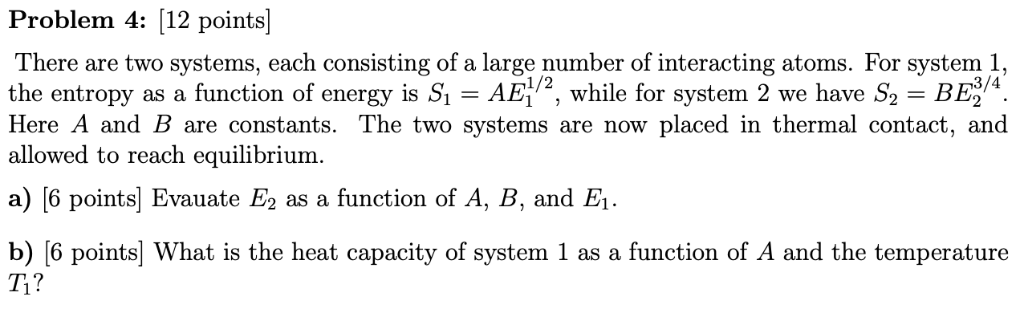Problem 4: [12 points] There are two systems, each consisting of a large number of interacting atoms. For system 1 the entropy as a function of energy is S,-AE1/2, while for system 2 we have S,-BEV4 Here A and B are constants. The two systems are now placed in thermal contact, and allowed to reach equilibrium. a) [6 points Evauate E2 as a function of A, B, and E1 b) [6 points] What is the heat capacity of system 1 as a function of A and the temperature T1?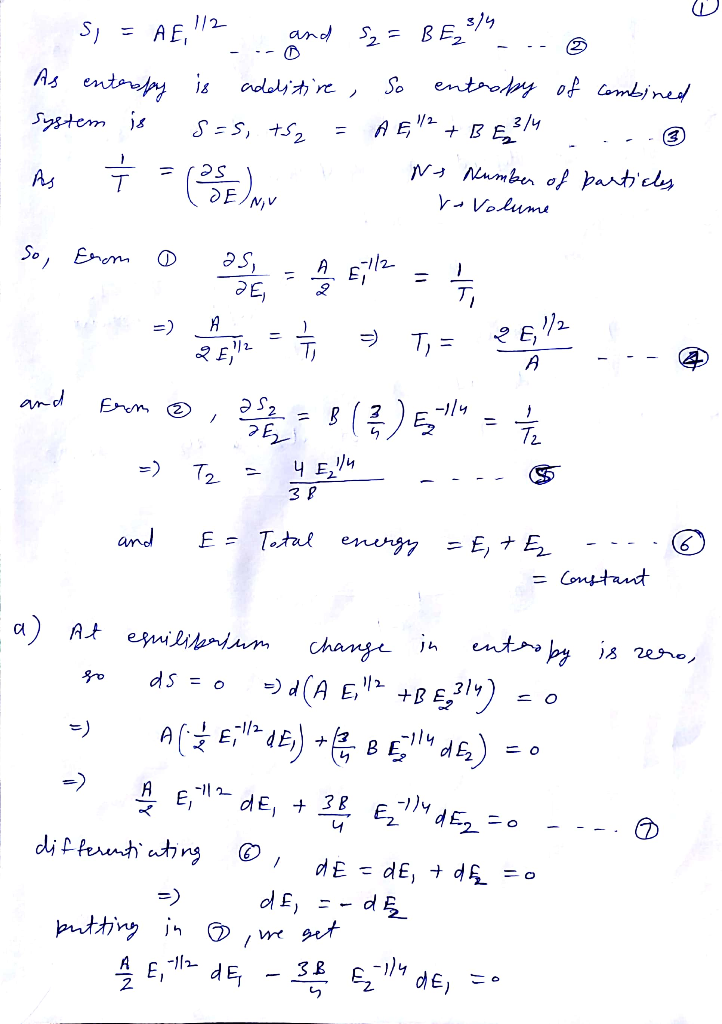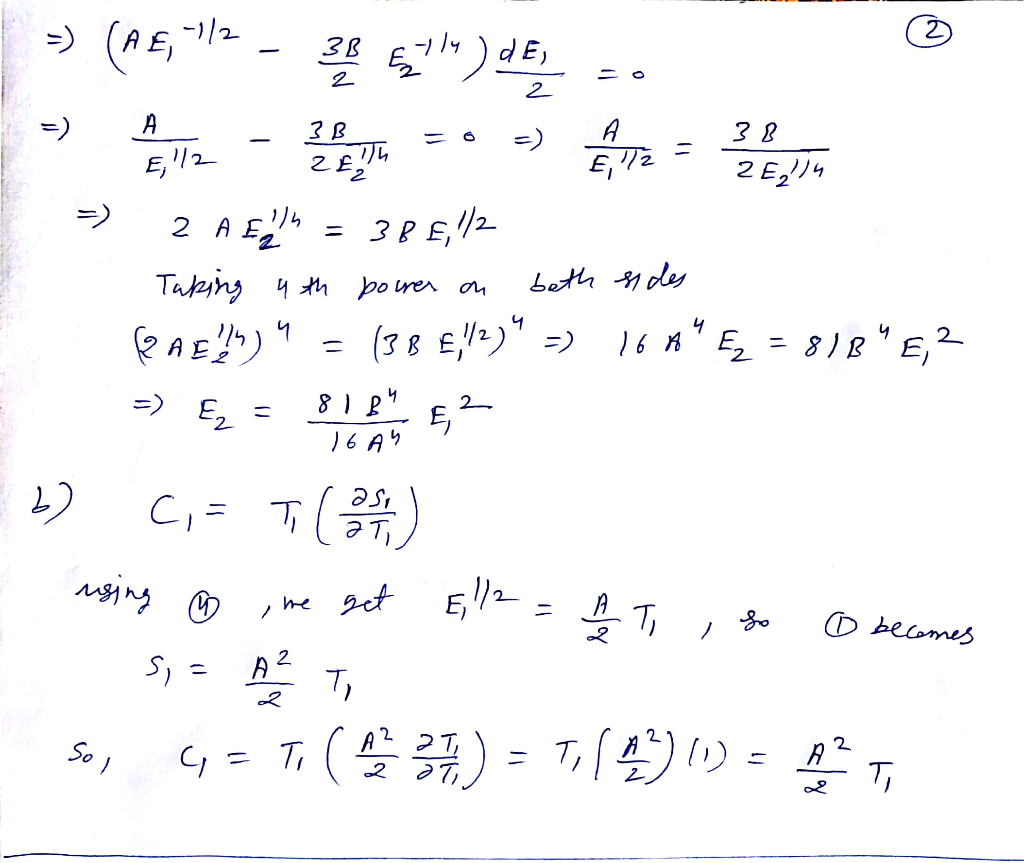#### Earn Coin

Coins can be redeemed for fabulous gifts.

Similar Homework Help Questions
• ### 3. Entropy of physical systems (a) Consider two interacting systems A and B. Calculate the number of microstates an...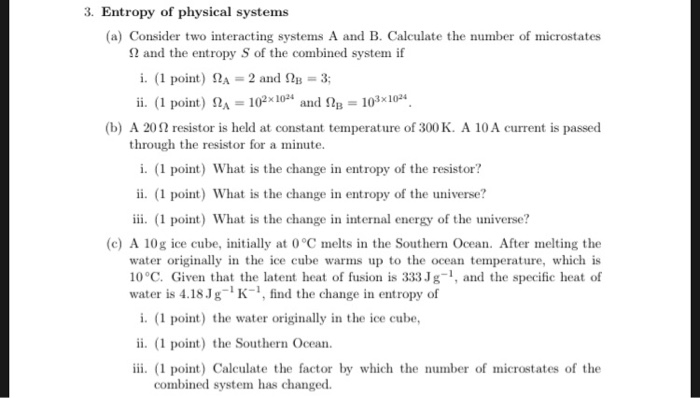3. Entropy of physical systems (a) Consider two interacting systems A and B. Calculate the number of microstates and the entropy S of the combined system if i. (1 point) ΩΑ-2 and Ω13 3; ii. (1 point) A0204 and 2B 103x104 (b) A 20 Ω resistor is held at constant temperature of 300 K. A 10 A current is passed through the resistor for a minute i. (1 point) What is the change in entropy of the resistor? ii. (1...

• ### Let's say we have 4 small interacting systems. I've already calculated the number of microstates for...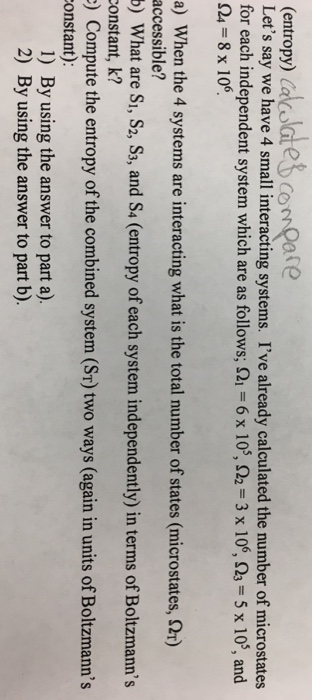Let's say we have 4 small interacting systems. I've already calculated the number of microstates for each independent system which are as follows; Ohm_1 = 6 Times 10^5, Ohm_2 = 3 Times 10^6, Ohm_3 = 5 Times 10^5, and Ohm_4 = 8 Times 10^6. a) When the 4 systems are interacting what is the total number of states (microstates, Ohm_T) accessible? b) What are S_1, S_2, S_3, and S_4 (entropy of each system independently) in terms of Boltzmann's Constant, k?...

• ### need help with thermodynamics A system consists of N weakly interacting particles, each of which can...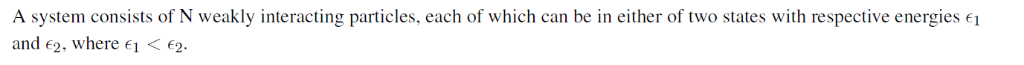need help with thermodynamics A system consists of N weakly interacting particles, each of which can be in either of two states with respective energies e and 2. where e1 2 1. Without explicit calculation, make a qualitative plot of the mean energy U the entropy S of the system as a function of its temperature T. What is in the limit of very low and very high temperatures? What is S in the limit of very low and very...

• ### Two vessels A and B each contain N molecules of the same ideal monatomic gas at the same pressure P. Initially, the two...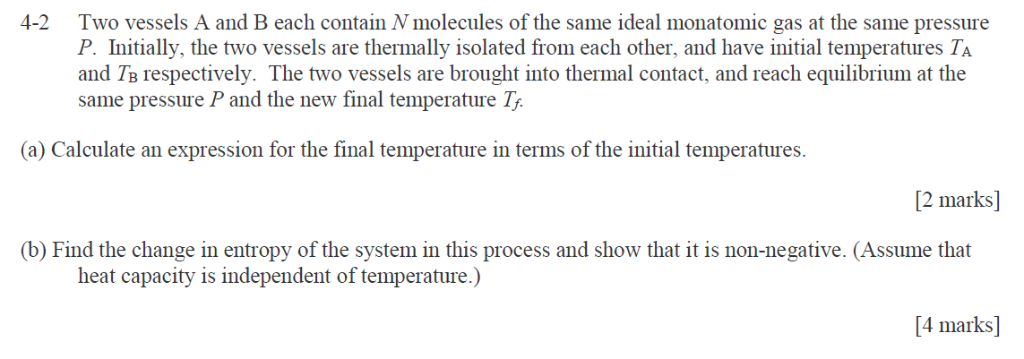Two vessels A and B each contain N molecules of the same ideal monatomic gas at the same pressure P. Initially, the two vessels are thermally isolated from each other, and have initial temperatures TA and Ta respectively. The two vessels are brought into thermal contact, and reach equilibrium at the same pressure P and the new final temperature 7, 4-2 (a) Calculate an expression for the final temperature in terms of the initial temperatures. [2 marks] (b) Find the...

• ### 2. Consider an isolated system consisting of a large number N of very weakly interacting localized...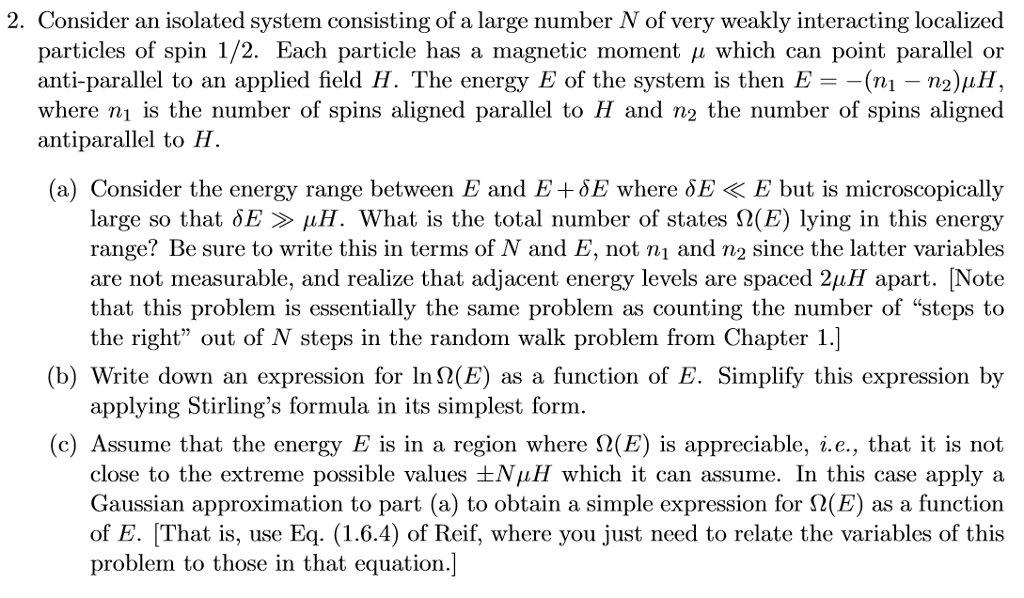2. Consider an isolated system consisting of a large number N of very weakly interacting localized particles of spin 1 2. Each particle has a rnagnetic mioment μ which can point parallel or anti-parallel to an applied field H. The energy E of the systern is then E =-(ni-n2):1H, antiparallel to H. (a) Consider the energy range between E and E+δΕ where δΕ < E but is microscopically large so that δΕ μΗ. What is the total number of states...

• ### Problem 3: (40 points) One-dimensional relativistic gas: Here we consider a non-interacting gas of N relativistic...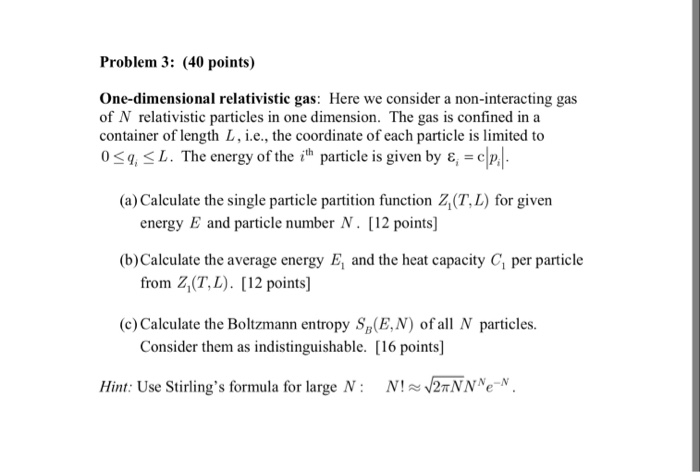Problem 3: (40 points) One-dimensional relativistic gas: Here we consider a non-interacting gas of N relativistic particles in one dimension. The gas is confined in a container of length L, i.e., the coordinate of each particle is limited to 0 <q < L. The energy of the ith particle is given by ε = c (a) Calculate the single particle partition function Z(T,L) for given energy E and particle number N. [12 points] (b) Calculate the average energy E and...

• ### 2. Interacting Spins (5 points each part, 30 points total). Two spins, each of which can...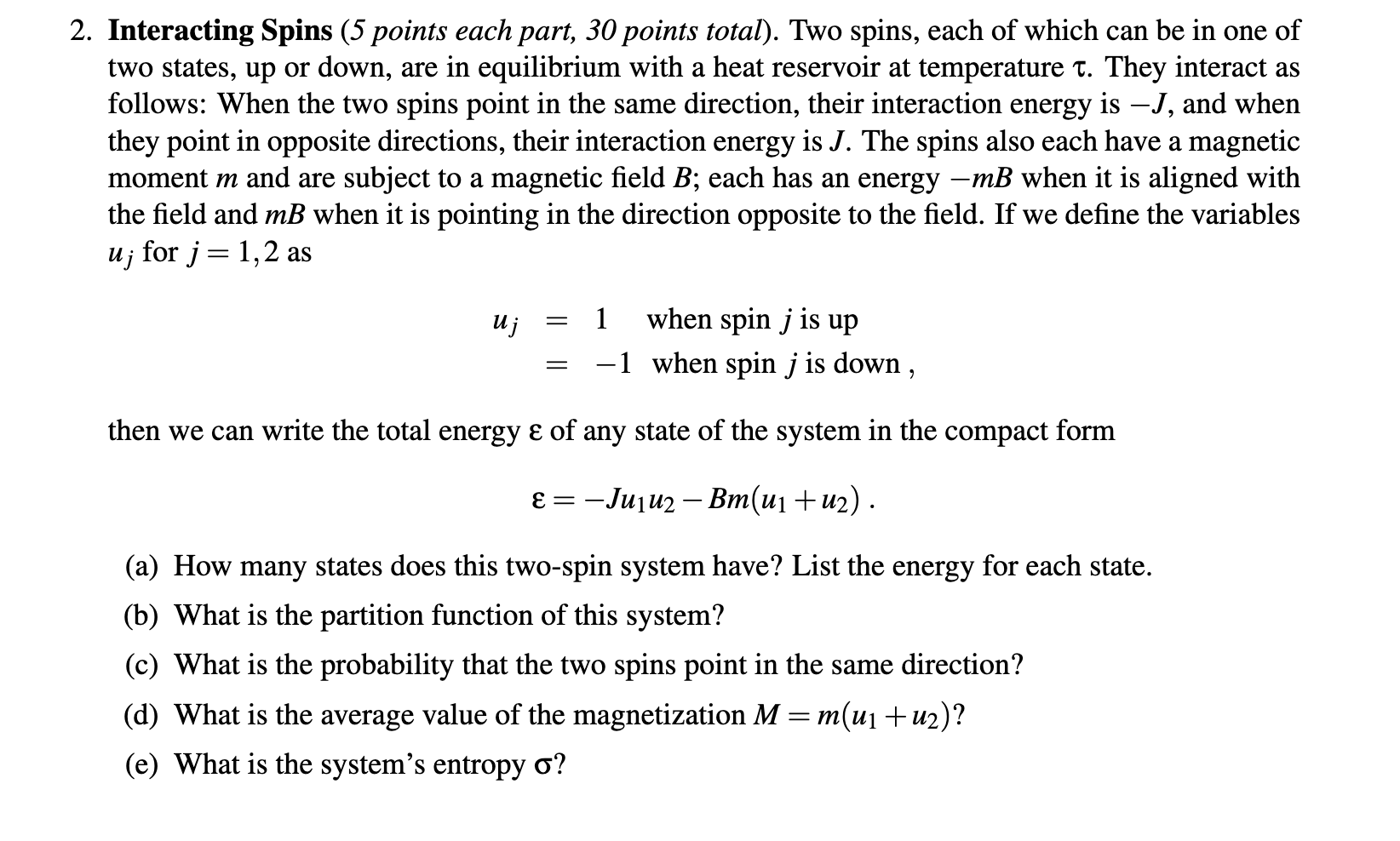2. Interacting Spins (5 points each part, 30 points total). Two spins, each of which can be in one of two states, up or down, are in equilibrium with a heat reservoir at temperature t. They interact as follows: When the two spins point in the same direction, their interaction energy is – J, and when they point in opposite directions, their interaction energy is J. The spins also each have a magnetic moment m and are subject to a...

• ### Generalize this problem: the two containers are at temperatures T1 and T2 (Kelvin) and heat Q...

Generalize this problem: the two containers are at temperatures T1 and T2 (Kelvin) and heat Q flows from container 1 to container 2. a. Derive a formula for the change in entropy ∆S (for the entire system) as a function of Q, T1 and T2. b. Show that ∆? ≥ 0 only if T1 ≥ T2.

• ### Answer a total of any THREE out of the four questions. Put the solution to each problem in a separate blue book and put the number of the problem and your name on the front of each book. If you su...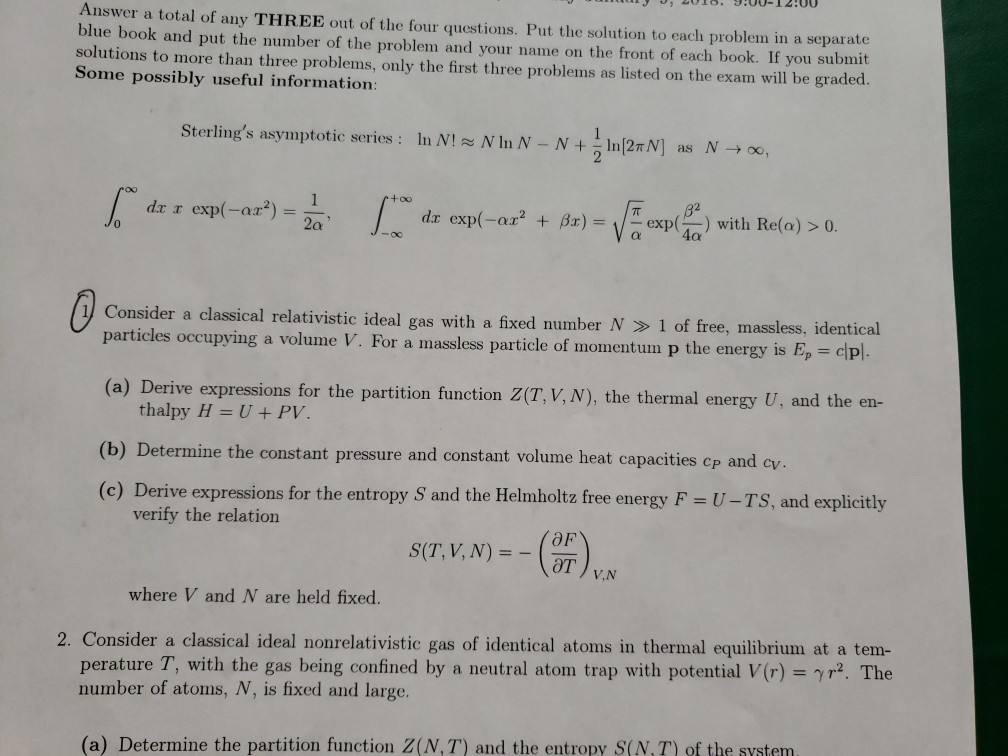Answer a total of any THREE out of the four questions. Put the solution to each problem in a separate blue book and put the number of the problem and your name on the front of each book. If you submit solutions to more than three problems, only the first three problems as listed on the exam will be graded. Some possibly useful information: Sterling's asymptotic series: N In N-N + 1 ln(2nN] In N! N → oo, as 2...

• ### Problem 8: Two amounts of salt water at different temperatures are brought into thermal contact with...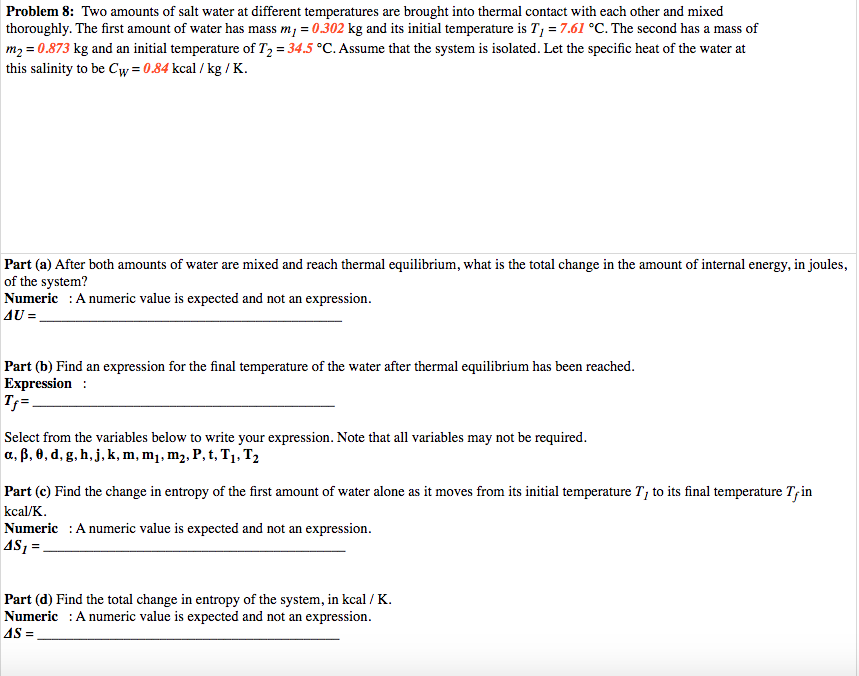Problem 8: Two amounts of salt water at different temperatures are brought into thermal contact with each other and mixed thoroughly. The first amount of water has mass m, = 0.302 kg and its initial temperature is T, = 7.61 °C. The second has a mass of m2 = 0.873 kg and an initial temperature of T2 = 34.5 °C. Assume that the system is isolated. Let the specific heat of the water at this salinity to be Cw=0.84 kcal...

Free Homework App